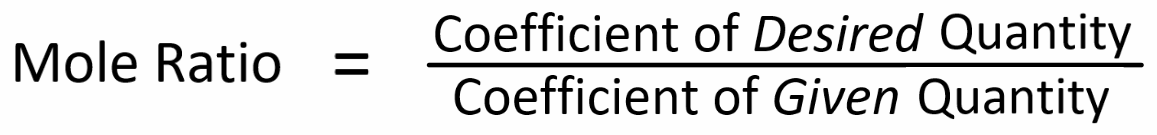# All Topics     |   Breslyn.org   |     Stoichiometry Topics

### Equation Stoichiometry

Equation stoichiometry is the culmination of many skills:

We put all of these skills together to solve mass to mass, mass to liters, and mass to molecules stoichoimetry problems.

### Solving Stoichiometry Problems:

In a typical problem we:
• convert grams, liters, or molecules given in the problem to moles.

• use the mole ratio to find moles of the desired quantity.

• convert moles of the desired quantity to grams, liters, or molecules. This is the answer!

More Help and Practice:

### Thinking Tools

It is helpful to visualize two mole maps connected by the mole ratio to solve stoichiometry problems:One way to find the the mole ratio is:We get the coefficients for the mole ratio from the balanced equation.

Printable Mole Map

Go To Top
Subscribe